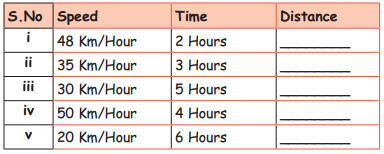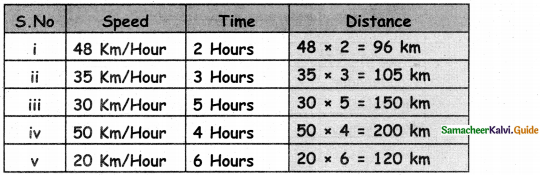Students can download 5th Maths Term 2 Chapter 5 Interconcept Ex 5.1 Questions and Answers, Notes, Samacheer Kalvi 5th Maths Guide Pdf helps you to revise the complete Tamilnadu State Board New Syllabus, helps students complete homework assignments and to score high marks in board exams.

## Tamilnadu Samacheer Kalvi 5th Maths Solutions Term 2 Chapter 5 Interconcept Ex 5.1

Question 1.Question 2.
i. Distance covered in 2 hours at the speed of 20 miles/hour:
20 × 2 = 40 miles

ii. Distance covered in 4 hours at the speed of 65 miles/hour:
65 × 4 = 260 miles

iii. Distance covered in 5 hours at the speed of 48 Km/hour:
48 × 5 = 240 km

iv. Distance covered in 6 hours at the speed of 80 Km/hour:
80 × 6 = 480 km

v. Distance covered in 3 hours at the speed of 42 Km/hour:
42 × 3 = 126 kmQuestion 3.
If Gopi ran 12 Hours at a speed of 14 Km/Hour, then how much distance did he ran?